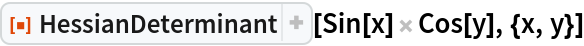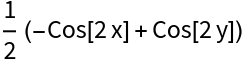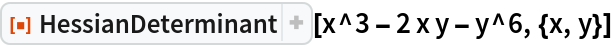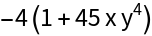Function Repository Resource:

# HessianDeterminant

Compute the Hessian determinant of a function with respect to a list of variables

Contributed by: Wolfram|Alpha Math Team
 ResourceFunction["HessianDeterminant"][expr,{var1, var2, …}] computes the determinant of the Hessian matrix of the expression expr with respect to the given variables.

## Examples

### Basic Examples (2)

Compute a Hessian determinant:

 In:=Out=Compute another Hessian determinant:

 In:=Out=## Publisher

Wolfram|Alpha Math Team

## Version History

• 3.0.0 – 06 September 2019
• 2.0.0 – 05 August 2019
• 1.0.0 – 12 April 2019